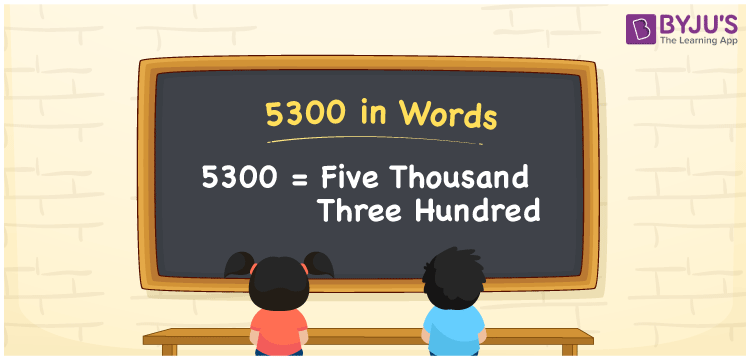# 5300 in Words

5300 in words is written as Five thousand three hundred. We can write the word form of the number 5300 using a place value chart effortlessly. However, we use the word form of 5300 in expressing a certain amount equivalent to 5300. Suppose a truck has 5300 packets of candies; we say it as “The truck contains five thousand three hundred packets of candies”. Let’s learn the process of converting the number 5300 into words.

 5300 in words Five thousand three hundred Five thousand three hundred in Numbers 5300

## 5300 in English Words

We generally write numbers in words using the English alphabet. Thus, we can express 5300 in English words as “Five thousand three hundred”.## How to Write 5300 in Words?

In this section, you will understand how to write 5300 in words using a four-column place value chart since it has four digits.

 Thousands Hundreds Tens Ones 5 3 0 0

Here, ones = 0, tens = 0, hundreds = 3, and thousands = 5.

Let us expand these digits as per their place values.

5 × Thousand + 3 × Hundred + 0 × Ten + 0 × One

= 5 × 1000 + 3 × 100 + 0 × 10 + 0 × 1

= 5000 + 300

= Five thousand + Three hundred

= Five thousand three hundred

Hence, 5300 in words = Five thousand three hundred.

As we know, 5300 is a natural number that precedes 5301 and succeeds 5299.

5300 in words – Five thousand three hundred

Is 5300 an odd number? – No

Is 5300 an even number? – Yes

Is 5300 a prime number? – No

Is 5300 a composite number? – Yes

Is 5300 a perfect square number? – No

Is 5300 a perfect cube number? – No

## Frequently Asked Questions on 5300 in Words

Q1

### How do you write 5300 in words?

Generally, we write the number 5300 in words as Five thousand three hundred.
Q2

### How to write 5300 rupees in words on a cheque?

On a cheque, we generally write 5300 rupees in words as Five thousand three hundred rupees only.
Q3

### Write the value of 2800 + 2500 in words.

2800 + 2500 = 5300 Therefore, the value of 2800 + 2500, i.e., 5300 in words is five thousand three hundred.